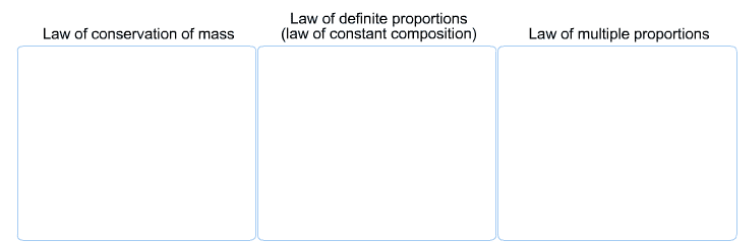# Problem: Match each observation to the law that it illustrates:The C:O mass ratio of one compound is exactly double that of another compound. The C:O mass ratio of a particular compound is the same regardless of the size or source of the sample.When 3g of carbon reacts with 8 g of oxygen, 11 g of carbon dioxide is produced.Burning 10 g of propane produces twice as much carbon dioxide burning 5 g of propane

###### FREE Expert Solution

Law of conservation of mass → all reactants are converted to products with nothing lost

Law of definite proportions → different samples of a pure compound always contain the same proportions of elements by mass

87% (45 ratings)###### Problem Details

Match each observation to the law that it illustrates:The C:O mass ratio of one compound is exactly double that of another compound.

The C:O mass ratio of a particular compound is the same regardless of the size or source of the sample.

When 3g of carbon reacts with 8 g of oxygen, 11 g of carbon dioxide is produced.

Burning 10 g of propane produces twice as much carbon dioxide burning 5 g of propane

Frequently Asked Questions

What scientific concept do you need to know in order to solve this problem?

Our tutors have indicated that to solve this problem you will need to apply the Law of Multiple Proportions concept. You can view video lessons to learn Law of Multiple Proportions. Or if you need more Law of Multiple Proportions practice, you can also practice Law of Multiple Proportions practice problems.